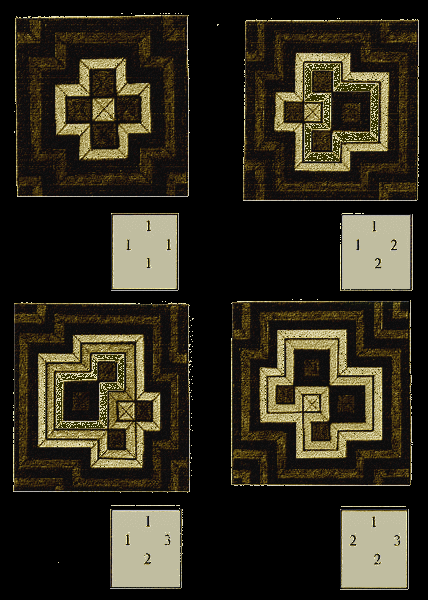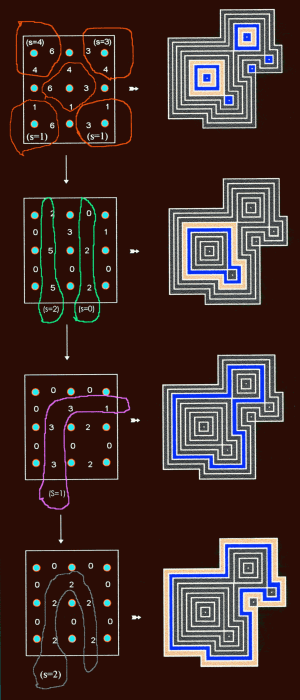# The principle of continued Subtraction

exampleIn this example six infinite bisectors intercross three by three. This results in 4 mutually independent length values in the system of bisectors (illustration a). These values dictate the number and shape of the strip-circuits which show up in the system (illustration b). At the one extremity of the value spectrum all four values are 1 (illustration c). In this case 5 squarely shaped circuits show up which, together, are encompassed by an infinite number of cross-like shaped circuits. If values higher than 1 are introduced, more circuits will arise within the space demarcated by the inner side of the first appearing crosslike shaped circuit and the outskirts of the 5 squarely shaped circuits .These circuits can be squarely shaped too, or they may take more complex forms. The relation between the introduced values and the arising circuit-shapes can be grasped in terms of 'continued subtraction'. The principle of continued Subtractioncontinued subtraction: In successive steps, certain values (s) are subtracted from the original values in the scheme, until all rest-values have reached zero. To determine these values (substrahends), in each step specific zones are selected from the overall value-scheme. In each of these zones, the lowest value is the subtrahend s . It is subtracted from all values in that zone. So in each zone at least one value reaches the level zero. The value of the subtrahend is equal to the number of strip-circuits that arises as a result of this part of the continued subtraction. The shape of the circuits which arise as a result of the subtractions in a certain step are specific for that step. It is related to the attributes of the zones selected in that step. Circuits that arise in later steps encompass circuits that arise in former steps.